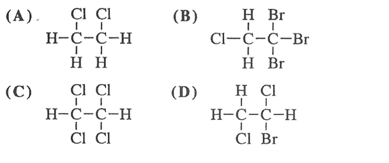# Problem: Which compound will have three peaks of equal height and three valleys of equal depth in a diagram of potential energy vs. angle of rotation for one complete rotation around the C-C bond?

###### FREE Expert Solution
97% (274 ratings)
###### Problem Details

Which compound will have three peaks of equal height and three valleys of equal depth in a diagram of potential energy vs. angle of rotation for one complete rotation around the C-C bond?Frequently Asked Questions

What scientific concept do you need to know in order to solve this problem?

Our tutors have indicated that to solve this problem you will need to apply the Dihedral Angle concept. If you need more Dihedral Angle practice, you can also practice Dihedral Angle practice problems.

What is the difficulty of this problem?

Our tutors rated the difficulty ofWhich compound will have three peaks of equal height and thr...as high difficulty.

What professor is this problem relevant for?

Based on our data, we think this problem is relevant for Professor Perks & Gierasch's class at UMBC.